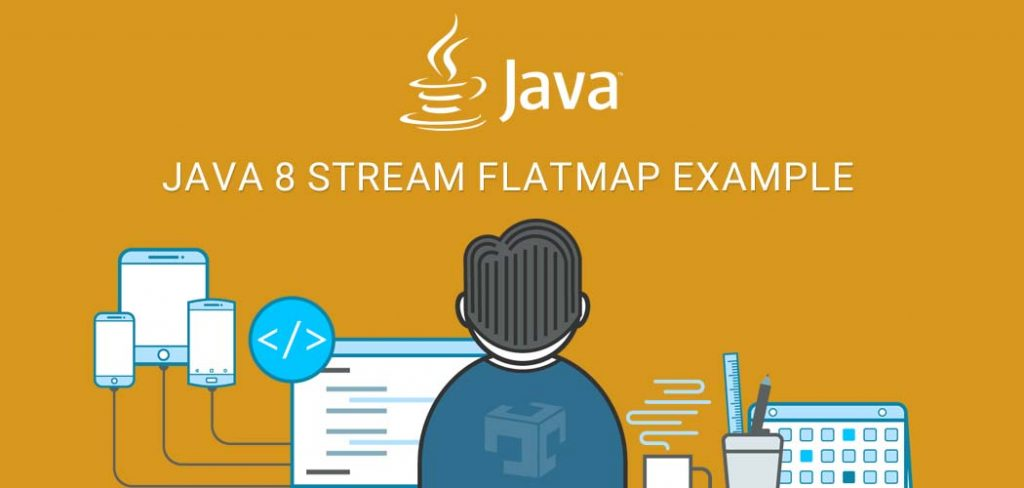# Java 8 Stream flatMap example

“flatMap name itself suggest that it is a combination of a map and a flat. It means that first we apply Function to our element and then flatten it”

Stream.map produce one output value for each input value whereas Stream.flatMap produce zero or more output value for each input value.

### How flatMap works:

{ {101,102}, {103,104}, {105,106} }     -> flatMap     -> {101,102,103,104,105,106}

{ {‘a’,’b’}, {‘c’,’d’}, {‘e’,’f’} }                     -> flatMap     -> {‘a’,’b’,’c’,’d’,’e’,’f’}

### flatMap one to many transformation:

flatMap applying a one-to-many transformation to the elements of the stream, and then flattening the resulting elements into a new stream.

Stream<String[]>             -> flatMap -> Stream<String>
Stream<int[]>                   -> flatMap -> Stream<int>
Stream<double[]>            -> flatMap -> Stream<double>
Stream<long[]>                -> flatMap -> Stream<long>
Stream<Set<String>>     -> flatMap -> Stream<String>
Stream<List<String>>    -> flatMap -> Stream<String>
Stream<List<Object>>   -> flatMap -> Stream<Object>

Now let’s discuss each flatMap transformation with example.

#### 1 ) Stream<String[]> -> flatMap ->Stream<String>

```String[][] data = new String[][]{{"java", "scala"}, {"python"}, {"C", "C++"}};
//Stream<String[]>
Stream<String[]> temp = Arrays.stream(data);
//Stream<String>
Stream<String> langStream = temp.flatMap(x -> Arrays.stream(x));
langStream.forEach(System.out::println);```
```java
scala
python
C
C++```

#### 2) Stream<Set<String>>  -> flatMap -> Stream<String>

##### POJO – Employee.java
```package com.javadevzone;
import java.util.ArrayList;
import java.util.Arrays;
import java.util.HashSet;
import java.util.Set;
/**
* Created by java developer zone on 5/21/2017.
*/
public class Employee {
private int no;
private String name;
private String designation;
private String gender;
private Set<String> languages;
public Employee(int no, String name, String designation, String gender , String [] languages) {
this.no = no;
this.name = name;
this.designation = designation;
this.gender = gender;
this.languages = new HashSet<>(Arrays.asList(languages));
}
}
//Getter , setter and toString

public static java.util.List<Employee> getEmployee() {
java.util.List<Employee> employees = new ArrayList<>();
employees.add(new Employee(1, "Bob", "Developer", "Male",new String[]{"java","scala"}));
employees.add(new Employee(2, "Joy", "Sr. Developer", "Male",new String[]{"java"}));
employees.add(new Employee(3, "John", "CEO", "Male",new String[]{"python","ruby"}));
employees.add(new Employee(4, "Bat", "Developer", "Male",new String[]{"scala"}));
employees.add(new Employee(5, "Jolly", "Developer", "Female",new String[]{"C","C++"}));
employees.add(new Employee(6, "Bobby", "Developer", "Female",new String[]{".Net","VB"}));
return employees;
}
}```

```List<Employee> employeeList = Employee.getEmployee();
List<String> collect =
employeeList.stream()
.map(x -> x.getLanguages())      //Stream<Set<String>>
.flatMap(x -> x.stream())   //Stream<String>
.distinct()
.collect(Collectors.toList());
collect.forEach(x -> System.out.println(x));
```
```java
scala
python
ruby
C++
C
.Net
VB```

#### 3 ) Stream<List<String>>  -> flatMap -> Stream<String>

```List<List<String>> stringList = new ArrayList<>();
List<String> jvmLanguage = new ArrayList<>();
List<String> otherLanguage = new ArrayList<>();
List<String> collect =
stringList.stream()
.flatMap(x -> x.stream())   //Stream<String>
.collect(Collectors.toList());

collect.forEach(x -> System.out.println(x));
```
```Java
Scala
C++
Ruby```

#### 4 ) Stream<int[]>  -> flatMap -> IntStream

```int[] intArray = {101, 102, 103};
//1. Stream<int[]>
Stream<int[]> streamArray = Stream.of(intArray);
//2. Stream<int[]> -> flatMap -> IntStream
IntStream intStream = streamArray.flatMapToInt(x -> Arrays.stream(x));
intStream.forEach(x -> System.out.println(x));```
```101
102
103```

#### 5 ) Stream<double[]> -> flatMap -> DoubleStream

```double[] intArray = {101.1d, 102.2d, 103.3d};
//1. Stream<double[]>
Stream<double[]> streamArray = Stream.of(intArray);
//2. Stream<double[]> -> flatMap -> DoubleStream
DoubleStream doubleStream = streamArray.flatMapToDouble(x -> Arrays.stream(x));
doubleStream.forEach(x -> System.out.println(x));```
```101.1
102.2
103.3```

#### 6 ) Stream<long[]> -> flatMap -> LongStream

```long[] intArray = {1000001L, 1000002L, 1000003L};
//1. Stream<long[]>
Stream<long[]> streamArray = Stream.of(intArray);
//2. Stream<long[]> -> flatMap -> LongStream
LongStream longStream = streamArray.flatMapToLong(x -> Arrays.stream(x));
longStream.forEach(x -> System.out.println(x));```
##### Output
```1000001
1000002
1000003```

Refer Stream , LongStream , IntStream , DoubleStream for more details.

### Source Code

You can also download source code of Java Stream FlatMap Example from our git repository.